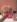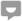# How to Solve a Series Circuit in Electronics

Knoji reviews products and up-and-coming brands we think you'll love. In certain cases, we may receive a commission from brands mentioned in our guides. Learn more.
A quick and easy guide to solve unknown values in a series circuit.

A series circuit, also known as a simple circuit, is a circuit in which there is only one path for current to follow. It can have resistors, inductors, capacitors, light bulbs, and other things connected, but as long as there is a single path from positive to negative in the circuit, the circuit is in series. See the figure below.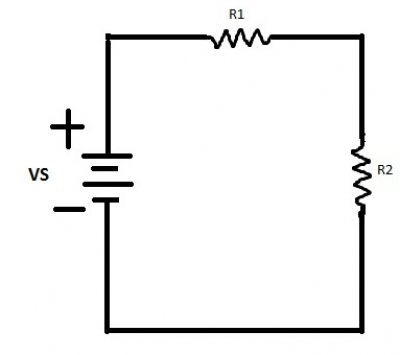According to Kirchoff’s Voltage Law, the sum of all voltages in a series circuit will add up to the source voltage (VS) (or, as some understand it, the sum of all voltages subtracted from the source voltage will be equal to zero). The reason behind this is because whatever voltage is fed into a series circuit (sometimes called the Voltage Rise) has to go somewhere. Every component in a circuit that has current flowing through it and a resistive value will have what’s known as a Voltage Drop. The relationship behind this law is referred to as Ohm’s Law, which is signified by the triangle below.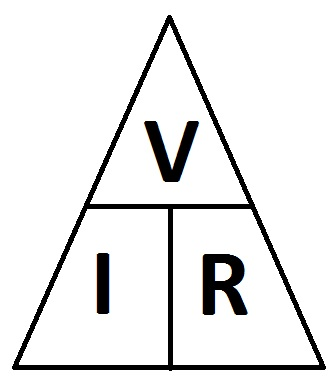The triangle means the following:

Voltage (V) / Current (I) = Resistance (R)

Voltage (V) / Resistance (R) = Current (I)

Resistance (R) * Current (I) = Voltage (V)

*Note: Voltage (V) can also be signified by an E.

If the sum of all voltages has to add up to the equivalent value of the source voltage, then that would mean that one of the values in Ohm’s Law would have to be a constant. In the case of the series circuit, the constant value would be that of the current. Whatever the value of the current is when it enters the circuit is the same value of the current at the ‘end’ of the circuit. Confused? See the diagram below.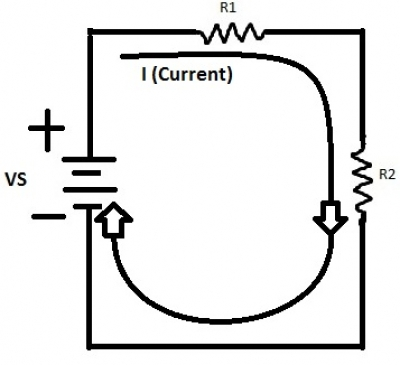With Ohm’s Law we can identify the theoretical value of each component in a series circuit. For example, if I have a 10 V battery connected to a series circuit with a resistor with a value of 100 ohms (ohms being the units for resistance), and we then proceed to connect another resistor of an unknown value to the circuit, we can calculate the value of the second resistor. We can also prove that the current going through the second resistor is equal to the current going through the first resistor. Don’t believe me? Take a look.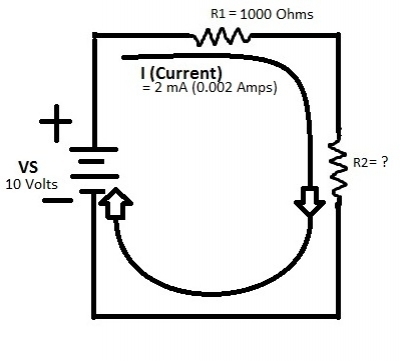First we need to find the voltage at R2. Since, according to Kirchoff’s Voltage Law, the voltages at the resistors would have to add up to the source voltage (V1 + V2 = VS), we can deduce that V2 = VS – V1. Of course, that would mean that we would need to know V1. No problem!

V1 = I * R1 (Ohm’s Law)

V1 = 2 milliAmps (mA) * 1000 Ohms

V1 = 2 Volts

If we know that V1 = 2 Volts, then that means that:

V2 = 10 V – 2 V = 8 Volts.

Everything is starting to come together now. We know that V2 is equal to 8 Volts, and since we have two known values at R2 (the voltage and the current), we can now find the value of the resistor.

R2 = V/I (Ohm’s Law)

R2 = 8 V / .002 A

R2 = 4000 Ohms

*Note: The values used in this circuit are unrealistic. They were only chosen to show a simple example.

Now that we know the values for both resistors and the voltages at each, we can prove that the current running through the circuit is the same value at every point.

I (Current) = V/R (Ohm’s Law)

I = 8 V / 4000 Ohms

I = 0.002 Amps (2 mA)

It’s a match to our original current!

Series circuits are used in a variety of simple electronics, such as in the book lights that you buy at the dollar store (open one up and take a look!). There are many applications for simple circuits, some of which would surprise you. For further reading on simple circuits and how they apply to electronics, see any textbook about the fundamentals of electronics. Various websites also provide easy explanations and experiments that anyone can understand and do. If you would like to read about some interesting electrical facts, see: Crazy Electrical Facts.  If you would like to learn about parallel circuits, see: How to Do Calculations for a Parallel Circuit.